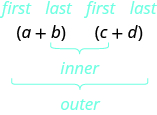## Summary: Performing Operations on Polynomials

Key Concepts
• Given multiple polynomials, how to add or subtract them to simplify the expressions

1. Combine like terms.
2. Simplify and write in standard form.
• Use the FOIL method for multiplying two binomials.
 Step 1. Multiply the First terms.Step 2. Multiply the Outer terms. Step 3. Multiply the Inner terms. Step 4. Multiply the Last terms. Step 5. Combine like terms, when possible.
• Multiplying Two Binomials: To multiply binomials, use the:
• Distributive Property
• FOIL Method
• Vertical Method
• Multiplying a Trinomial by a Binomial: To multiply a trinomial by a binomial, use the:
• Distributive Property
• Vertical Method

## Contribute!

Did you have an idea for improving this content? We’d love your input.